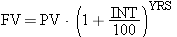Complete the program below so that it prompts for an initial amount of money (present value or PV) to deposit into a certificate of deposit (CD), the interest rate (INT) for the CD and the number of years (YRS) the CD will grow (until maturity). Compute and print out the future value of the CD after the given number of years has passed. You should use the following formula for future value (FV):Use the Math.pow() method shown in section 2.5.3.

While the number of years may be an integer, both the amount of money to be deposited and the interest rate should be double variables. The result (FV) should be printed out to two decimal places.

Complete the following file: